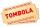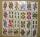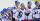# Pages counting

There are pages numbered from 2 to 104 in the book. How many digits have to be printed to number the pages?

Result

n =  203

#### Solution:

$n = 1 \cdot \ (10-2) + 2 \cdot \ (100-10)+3 \cdot \ (104-100+1) = 203$

Our examples were largely sent or created by pupils and students themselves. Therefore, we would be pleased if you could send us any errors you found, spelling mistakes, or rephasing the example. Thank you!

Leave us a comment of this math problem and its solution (i.e. if it is still somewhat unclear...):Be the first to comment!#### Following knowledge from mathematics are needed to solve this word math problem:

See also our variations calculator. Would you like to compute count of combinations?

## Next similar math problems:

1. Boys and girlsThere are eight boys and nine girls in the class. There were six children on the trip from this class. What is the probability that left a) only boys b) just two boys
2. RaffleThere are 200 draws in the raffle, but only 20 of them win. What is the probability of at least 4 winnings for a group of people who have bought 5 tickets together?
3. ClassroomOf the 26 pupils in the classroom, 12 boys and 14 girls, four representatives are picked to the odds of being: a) all the girls b) three girls and one boy c) there will be at least two boys
4. Families 2There are 729 families having 6 children each. The probability of a girl is 1/3 and the probability of a boy is 2/3. Find the the number of families having 2 girls and 4 boys.
5. Boys and girlsThere are 11 boys and 18 girls in the classroom. Three pupils will answer. What is the probability that two boys will be among them?
6. Three-digit numbersHow many three-digit numbers are from the numbers 0 2 4 6 8 (with/without repetition)?
7. CardsFrom a set of 32 cards we randomly pull out three cards. What is the probability that it will be seven king and ace?
8. Three redsWhat is the probability that when choosing 3 carats from seven carats, all 3 reds will be red?
9. Word MATEMATIKAHow many words can be created from the word MATEMATIKA by changing the order of the letters, regardless of whether or not the words are meaningful?
10. SalamiHow many ways can we choose 5 pcs of salami if we have 6 types of salami for 10 pieces and one type for 4 pieces?
11. Combinations of sweatersI have 4 sweaters two are white, 1 red and 1 green. How many ways can this done?
12. Three workplacesHow many ways can we divide nine workers into three workplaces if they need four workers in the first workplace, 3 in the second workplace and 2 in the third?
13. Sum or productWhat is the probability that two dice fall will have the sum 7 or product 12?
14. STRESSED wordEach letter in STRESSED is printed on identical cards, one letter per card and assembled in random order. Calculate the probability that the cards spell DESSERTS when assembled.
15. WordWhat is the probability that a random word composed of chars E, Y, G, E, R, O, M, T will be the GEOMETRY?
16. Hockey playersAfter we cycle five hockey players sit down. What is the probability that the two best scorers of this crew will sit next to each other?
17. Dices throwsWhat is the probability that the two throws of the dice: a) Six falls even once b) Six will fall at least once